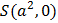# Ifand the equationrepresents an ellipse, thenbelongs to the interval a)b)c)d)## Question ID - 102695 :- Ifand the equationrepresents an ellipse, thenbelongs to the interval a)b)c)d)3537

(c)

The equationrepresents an ellipse having foci atandand major axis 3. Ifis the eccentricity of this ellipse, thenBut,andandBut,Therefore,Next Question :
 A gas will approach ideal behaviour at a) Low temperature and low pressure b) Low temperature and high pressure c) High temperature and low pressure d) High temperature and high pressure